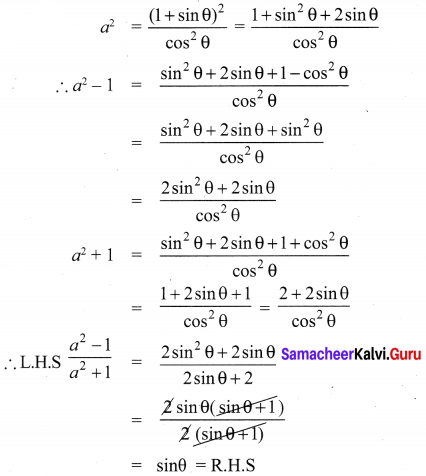## Tamilnadu Samacheer Kalvi 10th Maths Solutions Chapter 6 Trigonometry Ex 6.1

10th Maths Exercise 6.1 Samacheer Kalvi Question 1.
Prove the following identities.
(i) cot θ + tan θ = sec θ cosec θ
(ii) tan4θ + tan2θ = sec4θ – sec2θ
Solution: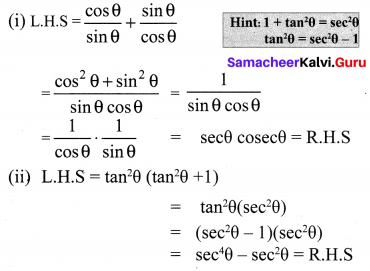10th Maths Trigonometry Exercise 6.1 Question 2.
Prove the following identities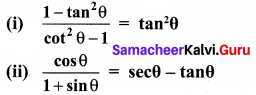Solution: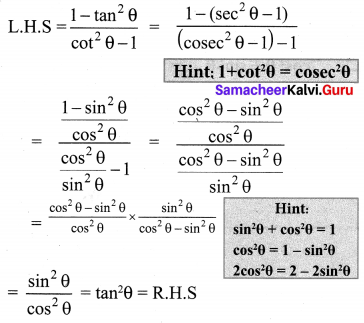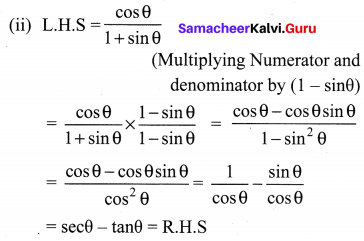Exercise 6.1 Class 10 Samacheer Kalvi Question 3.
Prove the following identities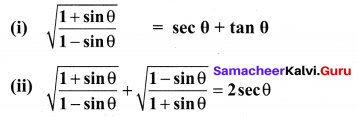Solution: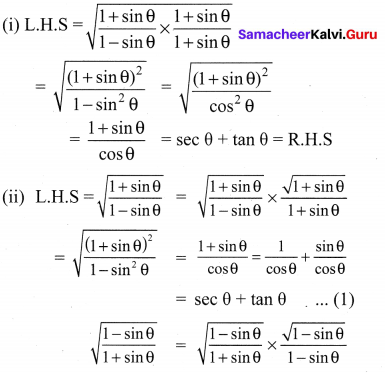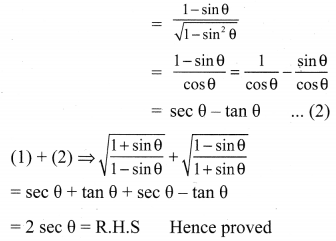10th Trigonometry Exercise 6.1 Question 4.
Prove the following identities
(i) sec6θ = tan6θ + 3tan2θ sec2θ + 1
(ii) (sinθ + secθ)2 + (cosθ + cosecθ)2
= 1 + (secθ + cosecθ)2
(i) L.H.S = sec6θ = (sec2θ)3 = (1 + tan2θ )3 = (tan2θ + 1)3
(a + b)3 = a3 + 3a2b + 3ab2 + b3
= (tan2θ)3 + 3(tan2θ)2 × 1 + 3 × tan2θ × 12 + 1
= tan6θ + 3tan2θ × (sec2θ – 1) + 3tan2θ + 1
= tan6θ + 3tan2θsec2θ – 3tan2θ + 3tan2θ +1
= tan6θ + 3tan2θ sec2θ + 1 = R.H.S

(ii) L.H.S = (sinθ + secθ )2 + (cosθ + cosecθ)2
= sin2θ + 2sinθ secθ + sec2θ + cos2θ + 2cosθ cosecθ+ cosec2θ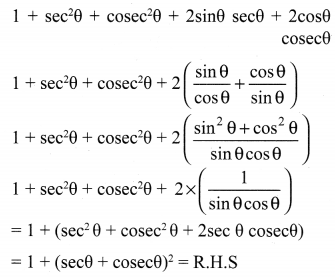Ex 6.1 Class 10 Samacheer Question 5.
Prove the following identities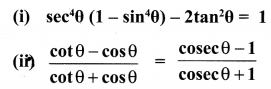Solution: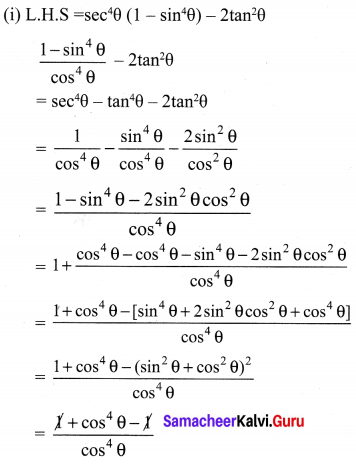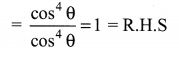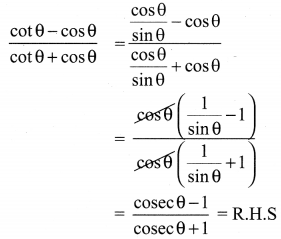10th Maths Exercise 6.1 Question 6.
Prove the following identities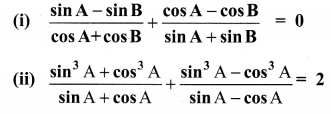Solution: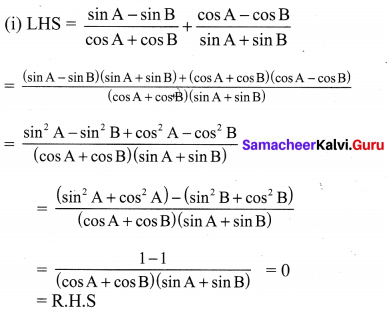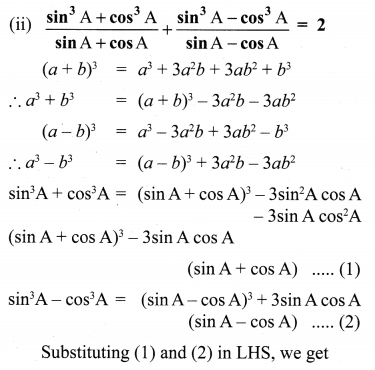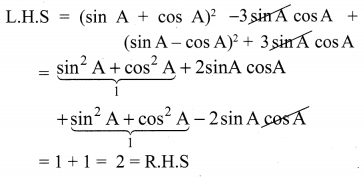Trigonometry Exercise 6.1 Question 7.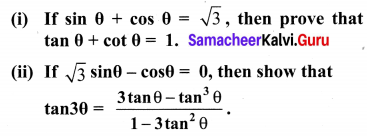Solution: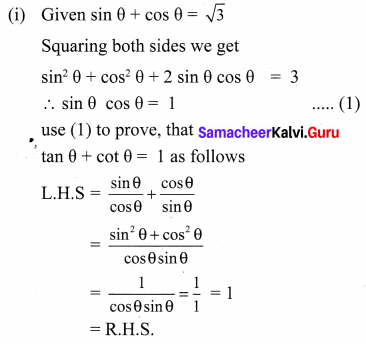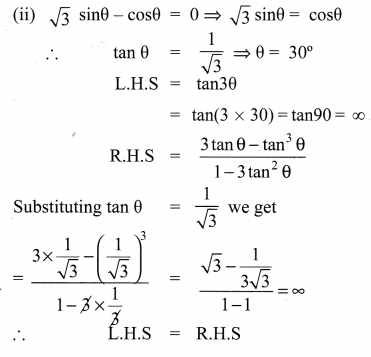10th Maths 6.1 Question 8.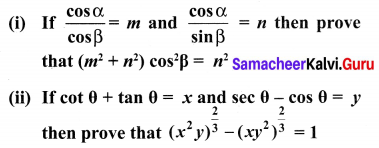Solution:
(i) LHS: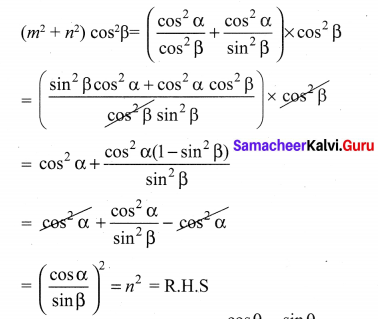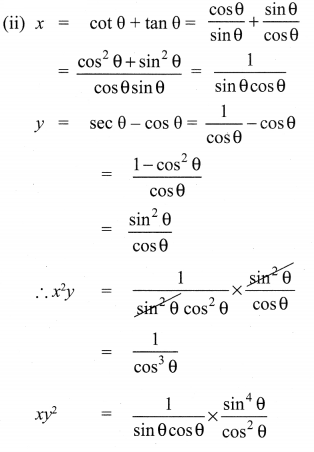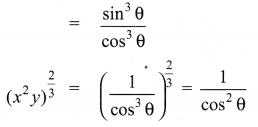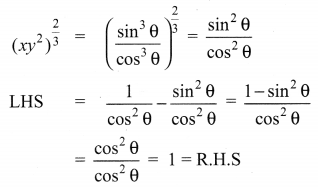10th Maths Exercise 6.1 In Tamil Question 9.
(i) If sinθ + cosθ = p and secθ + cosecθ = q then prove that q(p2 – 1) = 2p
(ii) If sinθ(1 + sin2θ) = cos2θ, then prove that cos6θ – 4cos4θ + 8cos2θ = 4
Solution: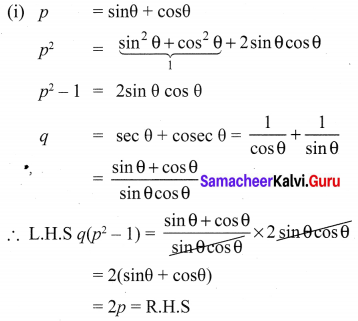(ii) Given sinθ(1 + sin2θ) = cos2θ
Sustitute sin2θ = 1 – co2θ and take cos θ = c
squaring (1) on bothsides we get
sin2θ(1 + sin2θ)2 = cos4θ
(1 – c2)(1 + 1 – c2) = c4
(1 – c2)(2 – c2)2 = c4
(1 – c2)(4 + c4– 4c2) = c4
4 + c4– 4c2 – 4c2 – c6 + 4c4 = c4
-c6 + 4c4 – 8c2 = -4
c6 – 4c4 + 8c2 = -4
ie cos 6θ – 4cos 4θ + 8cos2θ = 4 = RHS
∴ Hence proved

10th Samacheer Kalvi Maths Trigonometry Question 10.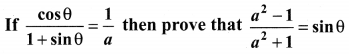Solution: